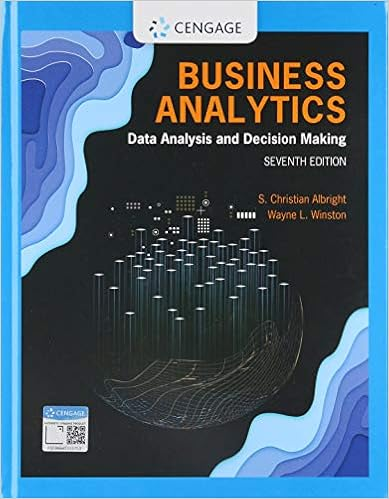# Stocks a and b have the following data the market

• Test Prep
• 33
• 98% (98) 96 out of 98 people found this document helpful

This preview shows page 12 - 15 out of 33 pages.

##### We have textbook solutions for you!
The document you are viewing contains questions related to this textbook.The document you are viewing contains questions related to this textbook.
Chapter 6 / Exercise 58
Business Analytics: Data Analysis & Decision Making
Albright/WinstonExpert Verified
40. Stocks A and B have the following data. The market risk premium is 6.0% and the risk-free rate is 6.4%. Assuming the stock market is efficient and the stocks are in equilibrium, which of the following statements is CORRECT? A B Beta 1.10 0.90 Constant growth rate 7.00% 7.00% a. Stock A must have a higher dividend yield than Stock B. b. Stock B's dividend yield equals its expected dividend growth rate. c. Stock B must have the higher required return. d. Stock B could have the higher expected return. e. Stock A must have a higher stock price than Stock B. ANS: A Statement a is true, because Stock A has a higher required return but the stocks have the same growth rate, so Stock A must have the higher dividend yield. Here are some calculations to demonstrate the point. r RF beta RP M r Stock A 6.40% + 1.10 × 6.00% = 13.00% B 6.40% + 0.90 × 6.00% = 11.80% Div. Yld. g r Stock A D 1 /P 0 + 7.00% = 13.00% D 1 /P 0 = r g = 6.00% B D 1 /P 0 + 7.00% = 11.80% D 1 /P 0 = r g = 4.80%
PTS: 1 DIF: Difficulty: Moderate OBJ: LO: 7-5 NAT: BUSPROG: Analytic STA: DISC: Stocks and bonds LOC: TBA TOP: Constant growth model: CAPM KEY: Bloom’s: Analysis MSC: TYPE: Multiple Choice: Conceptual NOT: Question may require calculations to find the correct answer. 41. A stock is expected to pay a dividend of \$0.75 at the end of the year. The required rate of return is r s = 10.5%, and the expected constant growth rate is g = 6.4%. What is the stock's current price?
##### We have textbook solutions for you!
The document you are viewing contains questions related to this textbook.The document you are viewing contains questions related to this textbook.
Chapter 6 / Exercise 58
Business Analytics: Data Analysis & Decision Making
Albright/WinstonExpert Verified
b. \$17.84 c. \$18.29 d. \$18.75 e. \$19.22 ANS: C D 1 \$0.75 r s 10.5% g 6.4% P 0 = D 1 /(r s g) \$18.29 PTS: 1 DIF: Difficulty: Easy OBJ: LO: 7-5 NAT: BUSPROG: Analytic STA: DISC: Stocks and bonds LOC: TBA TOP: Constant growth valuation KEY: Bloom’s: Application MSC: TYPE: Multiple Choice: Problem 42. A stock just paid a dividend of D 0 = \$1.50. The required rate of return is r s = 10.1%, and the constant growth rate is g = 4.0%. What is the current stock price?
PTS: 1 DIF: Difficulty: Easy OBJ: LO: 7-5 NAT: BUSPROG: Analytic STA: DISC: Stocks and bonds LOC: TBA TOP: Constant growth valuation KEY: Bloom’s: Application MSC: TYPE: Multiple Choice: Problem 43. A share of Lash Inc.'s common stock just paid a dividend of \$1.00. If the expected long-run growth rate for this stock is 5.4%, and if investors' required rate of return is 11.4%, what is the stock price?
P 0 = D 1 /(r s g) \$17.57 PTS: 1 DIF: Difficulty: Easy OBJ: LO: 7-5 NAT: BUSPROG: Analytic STA: DISC: Stocks and bonds LOC: TBA TOP: Constant growth valuation KEY: Bloom’s: Application MSC: TYPE: Multiple Choice: Problem
•••